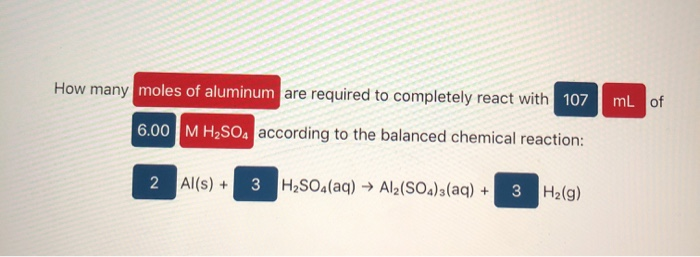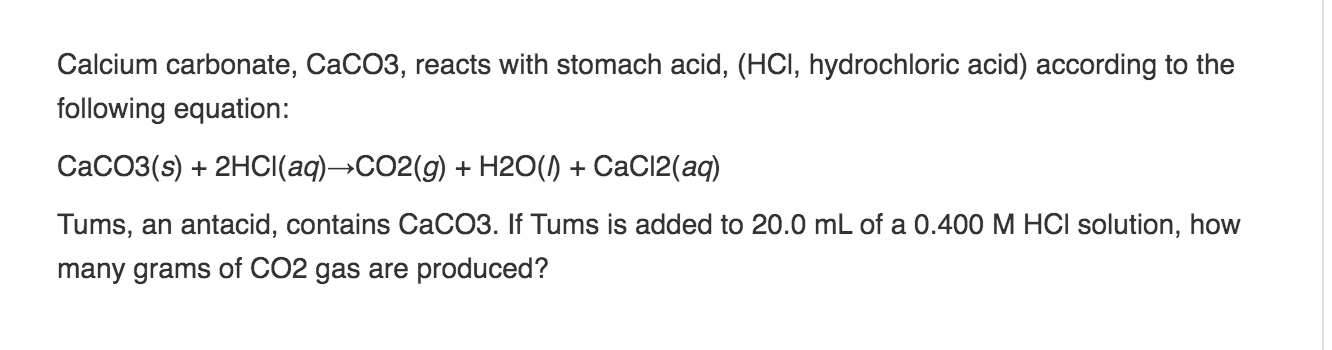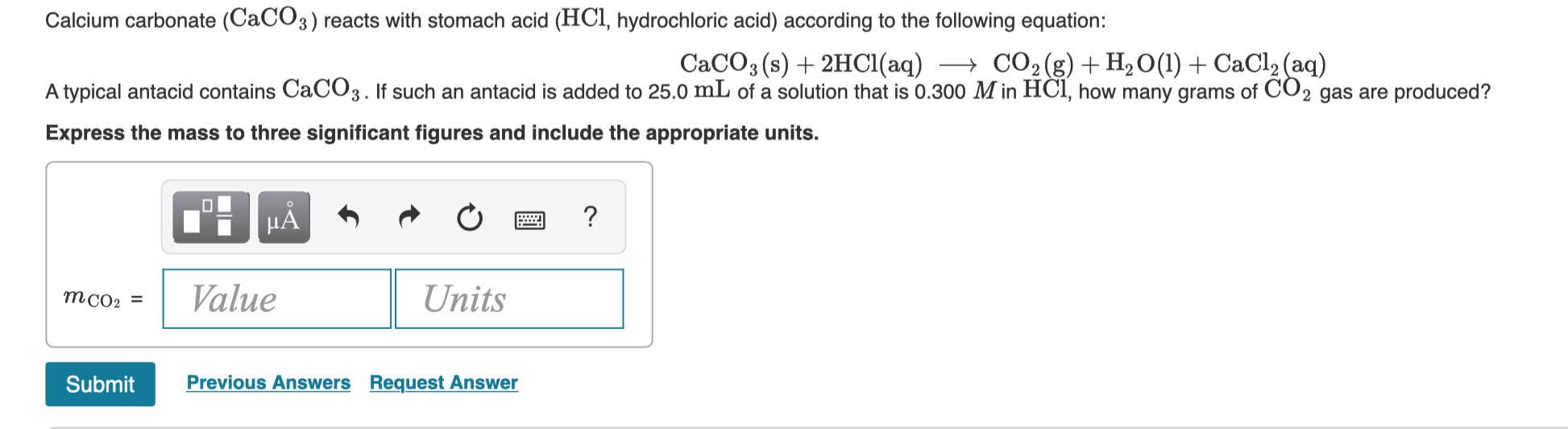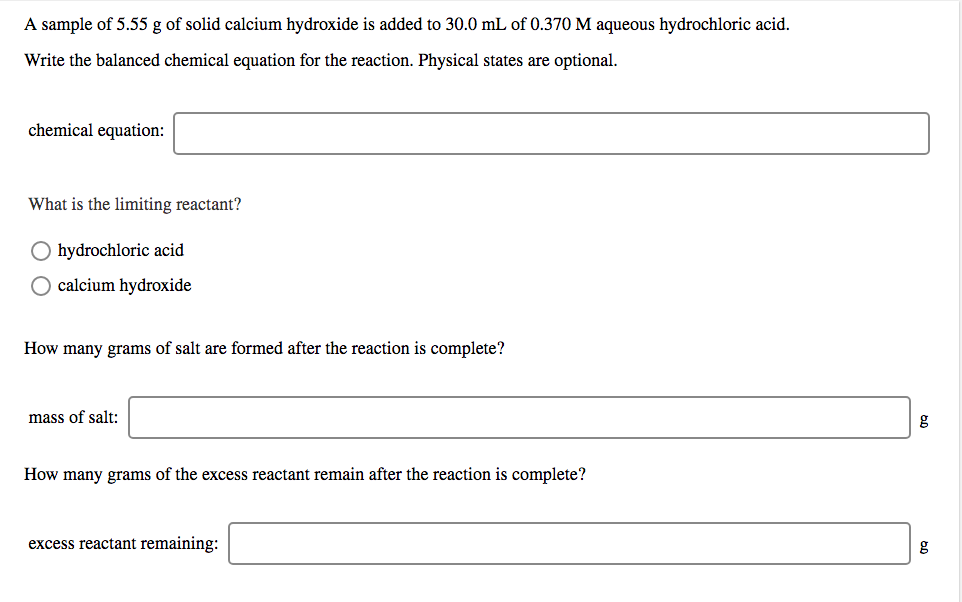# If 0.370 mol of solid CaCO3 and 940 mL of 0.702 M aqueous H2SO4 are reacted...

 If 0.370 mol of solid CaCO3 and 940 mL of 0.702 M aqueous H2SO4 are reacted stoichiometrically according to the balanced equation, how many moles of solid CaSO4 are produced? H2SO4(aq) + CaCO3(s) → CO2(g) + CaSO4(s) + H2O(l)

molarity of H2SO4 = number of moles of H2SO4 / volume of solution in L

0.702 = number of moles of H2SO4 / 0.940 L

number of moles of H2SO4 = 0.702 * 0.940 = 0.660 mole

from the balanced eqaution we can say that

1 mole of CaCO3 requires 1 mole of H2SO4 so

0.370 mole of CaCO3 will require 0.370 mole of H2SO4

but we have 0.660 mole of H2SO4 so H2SO4 is excess reactant

CaCO3 is limiting reactant

1 mole of CaCO3 produces 1 mole of CaSO4 so

0.370 mole of CaCO3 will produce 0.370 mole of CaSO4

1 mole of CaSO4 = 136.14 g

0.370 mole of CaSO4 = 50.4 g

Therefore, the mass of CaSO4 produced will be 50.4 g

#### Earn Coin

Coins can be redeemed for fabulous gifts.

Similar Homework Help Questions
• ### How many moles of aluminum are required to completely react with 107 mL of 6.00 M H2SO4 according to the balanced...How many moles of aluminum are required to completely react with 107 mL of 6.00 M H2SO4 according to the balanced chemical reaction: 2 Al(s) + 3 H2SO4(aq) → Al2(SO4)3(aq) + 3 H2(g)How many moles of AgI will be formed when 75.0 ml of 0.300 M AgNO3 completely reacted according to the balanced chemical reaction: 2 AgNO3(aq) + Cal2(aq) + 2 Agl(s) + Ca(NO3)2(aq)

• ### Calcium carbonate (CaCO3) reacts with stomach acid (HCl, hydrochloric acid) according to the following equation: CaCO3(s)+2HCl(aq)⟶CO2(g)+H2O(l)+CaCl2(aq)...

Calcium carbonate (CaCO3) reacts with stomach acid (HCl, hydrochloric acid) according to the following equation: CaCO3(s)+2HCl(aq)⟶CO2(g)+H2O(l)+CaCl2(aq) Tums, an antacid, contains CaCO3. If Tums is added to 10.0 mL of a solution that is 0.400 M in HCl, how many grams of CO2 gas are produced?

• ### If 770 mL of 0.701 M aqueous HClO4 reacts stoichiometrically according to the balanced equation, how...

If 770 mL of 0.701 M aqueous HClO4 reacts stoichiometrically according to the balanced equation, how many milliliters of 0.574 M aqueous Ba(OH)2are required? Ba(OH)2(aq) + 2HClO4(aq) ? Ba(ClO4)2(aq) + 2H2O(l)

• ### Calcium carbonate, CaCO3, reacts with stomach acid, (HCI, hydrochloric acid) according to the following equation: CaCO3(s)...Calcium carbonate, CaCO3, reacts with stomach acid, (HCI, hydrochloric acid) according to the following equation: CaCO3(s) + 2HCl(aq)-CO2(g) + H2O(1) +CaCl2(aq) Tums, an antacid, contains CaCO3. If Tums is added to 20.0 mL of a 0.400 M HCl solution, how many grams of CO2 gas are produced?

• ### Calcium carbonate (CaCO3) reacts with stomach acid (HCl, hydrochloric acid) according to the following equation: CaCO3(s)...Calcium carbonate (CaCO3) reacts with stomach acid (HCl, hydrochloric acid) according to the following equation: CaCO3(s) + 2HCl(aq) + CO2(g) + H2O(l) + CaCl2(aq) A typical antacid contains CaCO3. If such an antacid is added to 25.0 mL of a solution that is 0.300 M in HCl, how many grams of CO2 gas are produced? Express the mass to three significant figures and include the appropriate units. TI MÃ + + + a ? Value Units MCO: = Submit Previous...

• ### How many mL of 0.572 M HCl are needed to dissolve 9.65 g of CaCO3?

How many mL of 0.572 M HCl are needed to dissolve 9.65 g of CaCO3?2HCl(aq) +CaCO3(s)  CaCl2(aq) + H2O(l) + CO2(g)________mL

• ### A 0.450 g sample of impure CaCO3(s) is dissolved in 50.0 mL of 0.150 M HCl(aq)...

A 0.450 g sample of impure CaCO3(s) is dissolved in 50.0 mL of 0.150 M HCl(aq) . The equation for the reaction is CaCO3(s)+2HCl(aq)⟶CaCl2(aq)+H2O(l)+CO2(g) The excess HCl(aq) is titrated by 9.05 mL of 0.125 M NaOH(aq) . Calculate the mass percentage of CaCO3(s) in the sample.

• ### A sample of 5.55 g of solid calcium hydroxide is added to 30.0 mL of 0.370...A sample of 5.55 g of solid calcium hydroxide is added to 30.0 mL of 0.370 M aqueous hydrochloric acid. Write the balanced chemical equation for the reaction. Physical states are optional. chemical equation: What is the limiting reactant? hydrochloric acid calcium hydroxide How many grams of salt are formed after the reaction is complete? mass of salt: How many grams of the excess reactant remain after the reaction is complete? excess reactant remaining: A sample of 5.55 g of...

• ### 1.How many grams of glucose (C6H12O6) are produced if 17.3 mol of H2O are reacted according...

1.How many grams of glucose (C6H12O6) are produced if 17.3 mol of H2O are reacted according to this balanced chemical equation? 6CO2 + 6H2O → C6H12O6 + 6O2 2. Nitroglycerin [C3H5(ONO2)3] is made by reacting nitric acid (HNO3) with glycerol [C3H5(OH)3] according to this reaction: C3H5(OH)3 + 3HNO3 → C3H5(ONO2)3 + 3H2O If 87.4 g of HNO3 is reacted with excess glycerol, what mass of nitroglycerin can be made? 3. Precipitation reactions, in which a solid (called a precipitate) is...

• ### 10.0 mL of 0.360 M sodium sulfide is reacted with 25.00 mL of 0.415 M silver...

10.0 mL of 0.360 M sodium sulfide is reacted with 25.00 mL of 0.415 M silver nitrate according to the chemical equation shown below. (Na2S= 78.05 g/mol, AgNO3= 169.91 g/mol, NaNO3= 85.00 g/mol, Ag2S=172.02 g/mol) Na?2?S (aq) + 2AgNO3? (aq) yields (arrow) 2NaNO?3? (aq) +Ag2S (s) Caculate the theorectical yield of silver sulfde in grams. State which reactant if any any is limiting and explain how you know this. After all the limiting reactant is used, how many moles of...